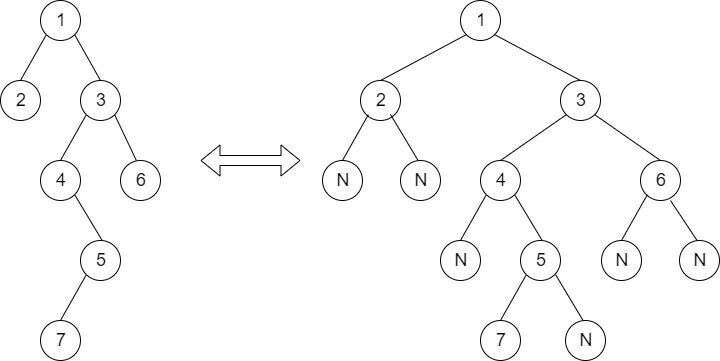Vertical Width of a Binary Tree
##### Submissions: 4310   Accuracy: 54.03%   Difficulty: Easy   Marks: 2

Given a Binary Tree of N nodes. The task is to find the vertical width of the tree.

The width of a binary tree is the number of vertical paths in that tree.The above tree contains 6 vertical lines which is the required vertical width of the tree.

Input:
First line of input contains the number of test cases T. For each test case, there will be only a single line of input which is a string representing the tree as described below:

1. The values in the string are in the order of level order traversal of the tree where, numbers denotes node values, and a character “N” denotes NULL child.

2. For example:For the above tree, the string will be: 1 2 3 N N 4 6 N 5 N N 7 N

Output:
For each test case, there will be a single line of output containing the vertical widht of the binary tree.

The task is to complete the function verticalWidth() which takes root as the only parameter, and returns the vertical width of the binary tree.

Constraints:
1 <= T <= 100
1 <= Number of nodes <= 103

Example:
Input:

2
1 2 3 4 5 6 7 N N N N N 8 N 9
1 2 3

Output:
6
3

Explanation:
See the above given figure.

#### ** For More Input/Output Examples Use 'Expected Output' option **

Contributor: Harshit Sidhwa
Author: harshitsidhwa

If you have purchased any course from GeeksforGeeks then please ask your doubt on course discussion forum. You will get quick replies from GFG Moderators there.